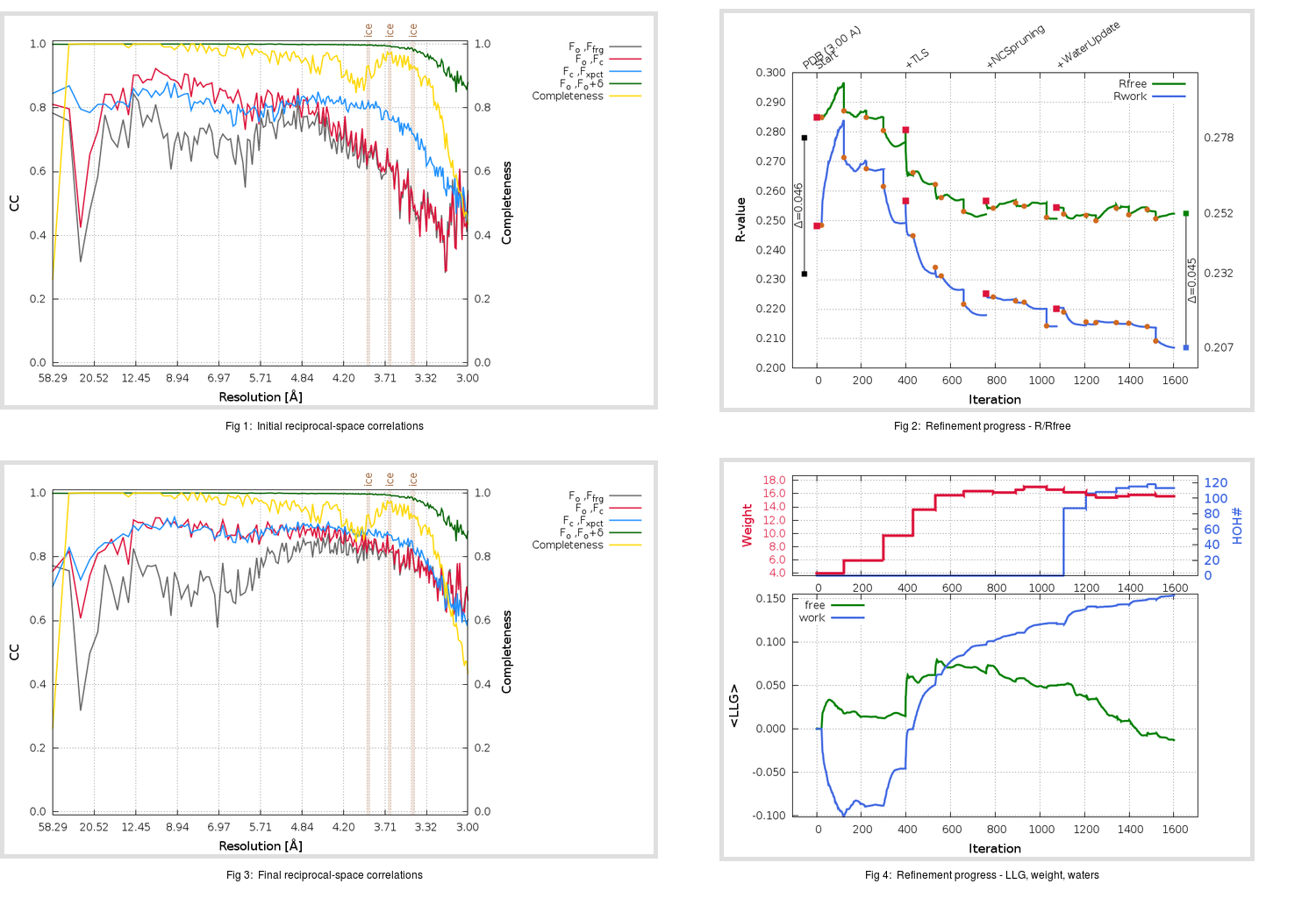Content:

```    Diffraction limits & principal axes of ellipsoid fitted to diffraction cut-off surface:
2.912         0.7503   0.0000  -0.6611       0.615 a* - 0.788 c*
3.001         0.0000   1.0000   0.0000       b*
3.195         0.6611   0.0000   0.7503       0.548 a* + 0.837 c*
```

## Deposited

` `
 Date deposited Date data collection Resolution R, Rfree 20110607 3.00 0.2300 0.2780

Molprobity (CCP4 7.0 version) summary:

```Ramachandran outliers =   4.31 %
favored =  82.55 %
Rotamer outliers      =   8.63 %
C-beta deviations     =     0
Clashscore            =  21.40
RMS(bonds)            =   0.0100
RMS(angles)           =   1.27
MolProbity score      =   3.24
Resolution            =   3.00
R-work                =   0.2300
R-free                =   0.2780
```

```Number of waters      =     0

<B> (all atoms) =   85.93 ( sd =   23.13 ) for      12544 non-hydrogen atoms
<B>   (protein) =   85.93 ( sd =   23.13 ) for      12540 non-hydrogen atoms
<B>     (water) =    0.00 ( sd =    0.00 ) for          0 non-hydrogen atoms
<B>    (others) =   94.50 ( sd =   12.92 ) for          4 non-hydrogen atoms

B min/max       (all non-hydrogen atoms) =   40.69 /  151.86
B min/max   (protein non-hydrogen atoms) =   40.69 /  151.86
B min/max     (water non-hydrogen atoms) =    0.00 /    0.00
B min/max     (other non-hydrogen atoms) =   82.82 /  114.86
```

## BUSTER (re-)refinement

` `

Molprobity (CCP4 7.0 version) summary:

```Ramachandran outliers =   1.31 %
favored =  93.99 %
Rotamer outliers      =  12.39 %
C-beta deviations     =     5
Clashscore            =   7.96
RMS(bonds)            =   0.0121
RMS(angles)           =   1.59
MolProbity score      =   2.67
Resolution            =   3.00
R-work                =   0.2070
R-free                =   0.2522
```

```Number of waters      =   113

<B> (all atoms) =  104.58 ( sd =   27.37 ) for      12657 non-hydrogen atoms
<B>   (protein) =  104.91 ( sd =   27.24 ) for      12540 non-hydrogen atoms
<B>     (water) =   68.27 ( sd =   13.79 ) for        113 non-hydrogen atoms
<B>    (others) =   98.17 ( sd =   18.11 ) for          4 non-hydrogen atoms

B min/max       (all non-hydrogen atoms) =   40.44 /  190.35
B min/max   (protein non-hydrogen atoms) =   57.64 /  190.35
B min/max     (water non-hydrogen atoms) =   40.44 /  121.26
B min/max     (other non-hydrogen atoms) =   74.32 /  120.08
```

Refinement progression:Results:

` `
 File Remark 3SCJ_aB_refine.01_04_refine.pdb.gz exact refinement commands are in header 3SCJ_aB_refine.01_04_refine.mtz.gz including original deposited data and several re-refinement map coefficients 3SCJ_aB_refine.01_04_BUSTER_model.cif.gz including any non-standard compound restraints 3SCJ_aB_refine.01_04_BUSTER_refln.cif.gz